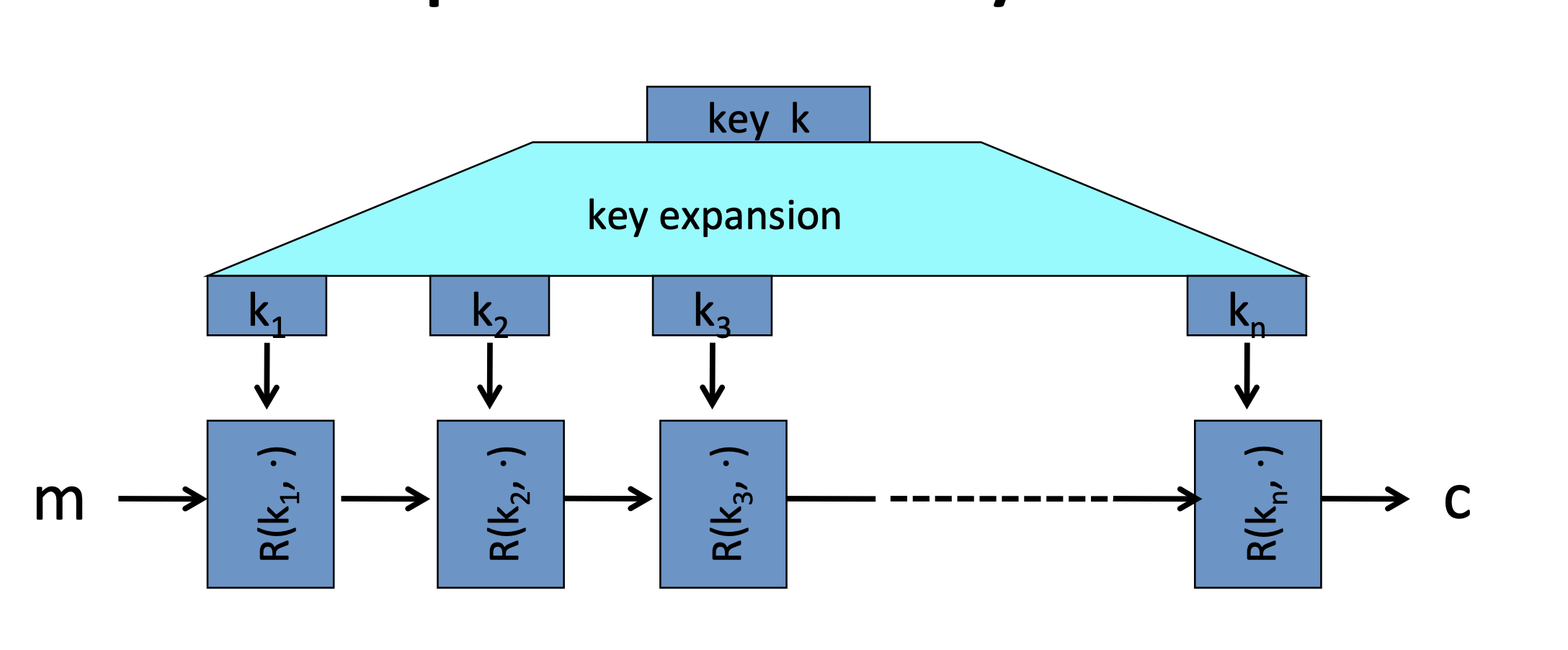# Overview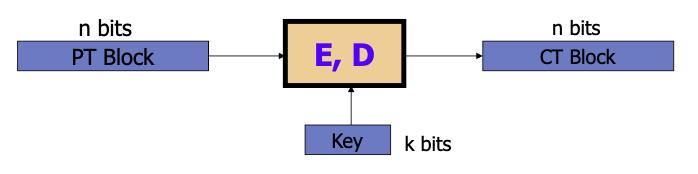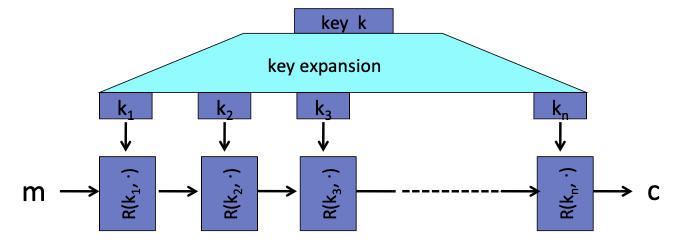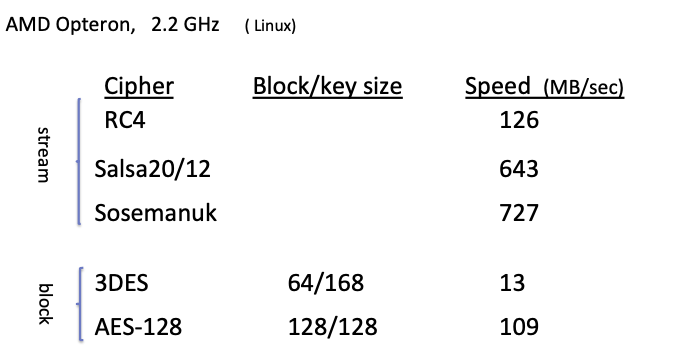## PRPs and PRFs

Pseudo Random Function(PRF):

defined over(K, X, Y): $F:K\times X \rightarrow Y$ such that exists “efficient” algorithm to evaluate F(k, x)

【伪随机函数定义在(K, X, Y)上，只要求是可计算的，没有要求是可逆的】

Pseudo Random Permutations(PRP):

defined over(K, X): $E:K\times X\rightarrow X$ such that:

1. Exists “efficient” deterministic algorithm to evaluate E(k, x)
2. The function E(k, ·) is one to one
3. Exists “efficient” inversion algorithm D(k, y)

【而伪随机置换定义在(K, X)上，除了要求是可计算的，还要求是一对一映射（单射），即存在可逆运算】

• AES: $K \times X \rightarrow X$ where K = X = $\{0, 1\}^{128}$

• 3DES: $K\times X \rightarrow X$ where X = $\{0, 1\}^{64}$ , K = $\{0, 1\}^{168}$

## Secure PRFs

$F: K \times X \rightarrow Y$ is a PRF.

• Funs[X, Y]: the set of all functions from X to Y

【X到Y的所有映射关系】

• $S_F={ F(k, \cdot)}$ s.t. $k\in K$ $\subseteq$ Funs[X, Y]

【确定k就确定了一种X到Y的映射关系】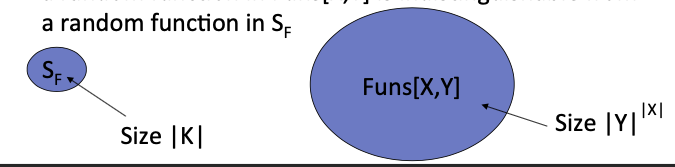Intuition: a PRF is secure if a random function in Funs[X, Y] is indistinguishable from a random function in $S_F$ .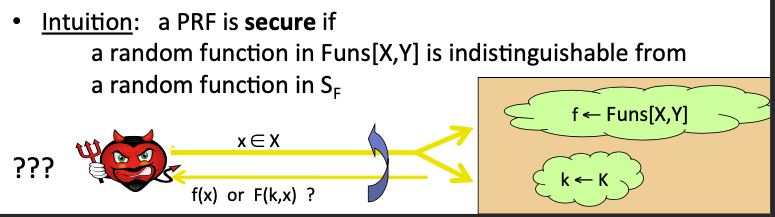## Secure PRPs/Block Ciphers

$E: K\times X\rightarrow Y$ is a PRP ( X = Y).

• Perms[X, Y]: the set of all one-to-one functions from X to Y

【X到Y的所有的一对一映射关系】

• $S_F={ E(k, \cdot)}$ s.t. $k\in K$ $\subseteq$ Perms[X, Y]

【确定k就确定了一种X到Y的一对一映射关系】

Intuition: a PRP is secure if a random function in Perms[X, Y] is indistinguishable from a random function in $S_F$ .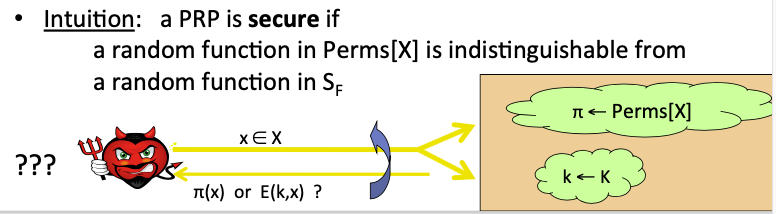## PRF $\rightarrow$ PRG

PRF的一个简单应用是把它变成一个PRG。

Let $F: K\times \{0, 1\}^n\rightarrow \{0, 1\}^n$ be a secure PRF.

The following $G: K\rightarrow \{0,1\}^{nt}$ is a secure PRF:

$$G(k) = F(k, 0)\mid\mid F(k, 1) \mid\mid \dots \mid\mid F(k, t-1)$$

# DES

Data Encryption Standard（DES）

DES的核心就是Feistel网络结构：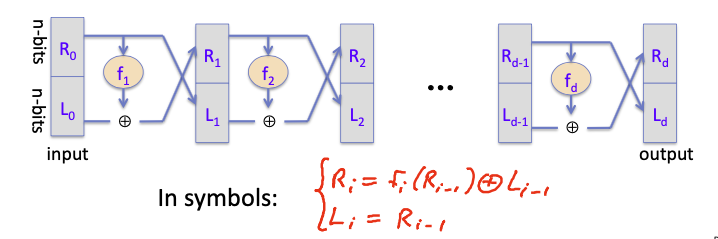• $R_i = f_i(R_{i-1})\oplus L_{i-1}$
• $L_i = R_{i-1}$

Claim: for all f1, …, fd: $\{0,1\}^n\rightarrow \{0,1\}$ , Feistel network $F: \{0,1\}^{2n}\rightarrow \{0,1\}^{2n}$ is invertible.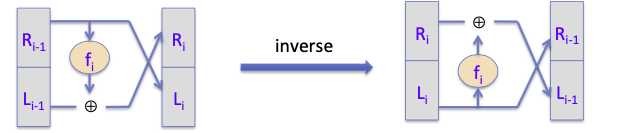• $R_{i-1} = L_i$
• $L_{i-1}=R_i\oplus f_i(L_i)$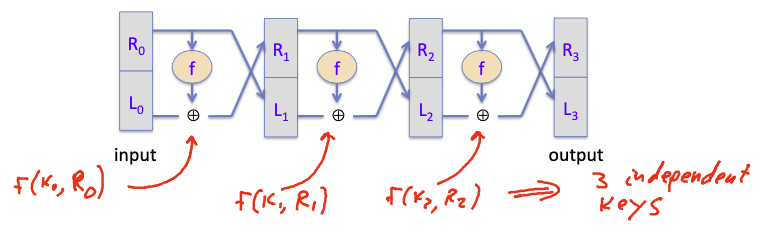## Luby-Rackoff’85 Thm

Thm:

$f:K\times \{0,1\}^n\rightarrow \{0,1\}^n$ a secure PRF

then, 3-round Feistel $F: K^3\times{0,1}^{2n}\rightarrow {0,1}^{2n}$ a secure PRP.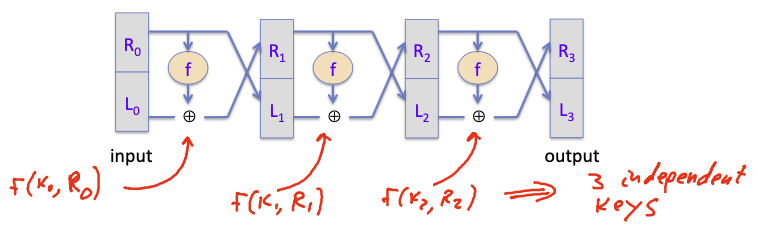## DES Structure

DES的核心是一个16轮的Feistel的网络。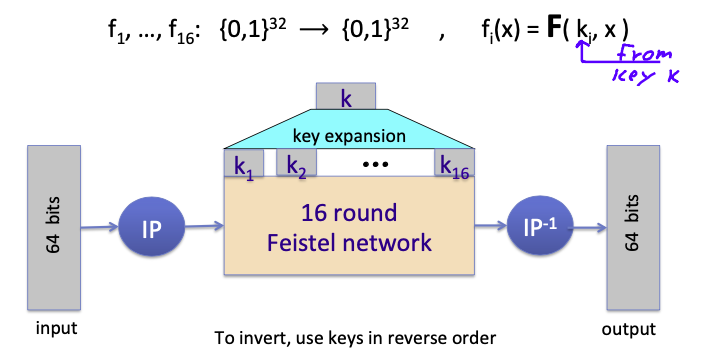DES的输入是64 bits块，通过一个初始置换（IP），该置换并不是为安全性服务的，只是DES标准里的要求。

### The function F(ki, x)

DES的轮函数结构如下图：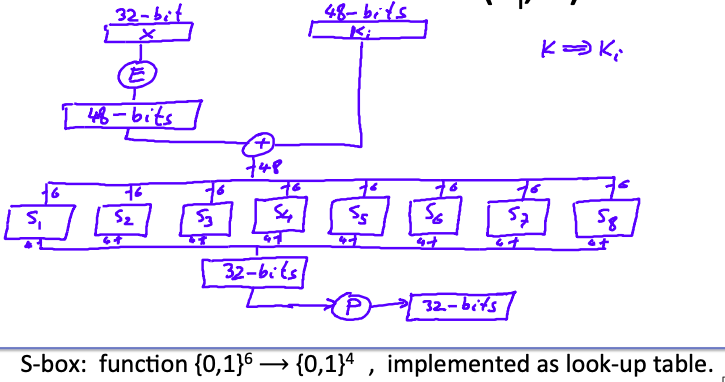1. 32 bits x通过expansion box，扩展为48 bits。扩展方式只是复制一些位。

2. 扩展后的x与轮密钥ki进行异或运算。

3. 异或运算后的结果为48 bits，再被划分为8组6 bits，每一组都通过一个S-box，每一个S-box实则是一个非线形映射 ${0,1}^6\rightarrow {0,1}^4$ ，在实现中往往使用查表的形式使用。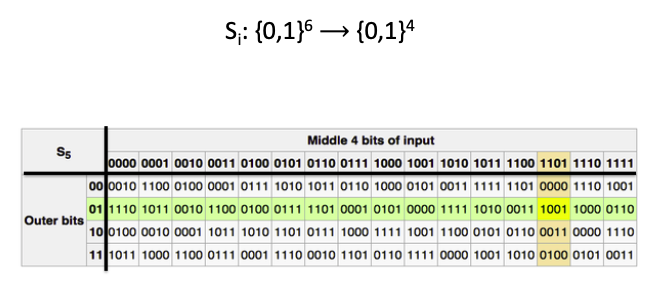4. 通过S-box后，又得到了32 bits，最后通过一个置换（Permutation），得到该轮的最终输出。

### S-box

S-box也是DES中最重要的一部分，因为他是DES中唯一的非线性部分，它保证了整个DES的非线形映射关系。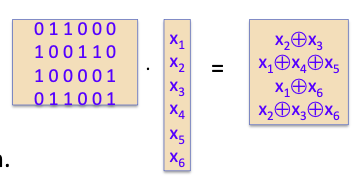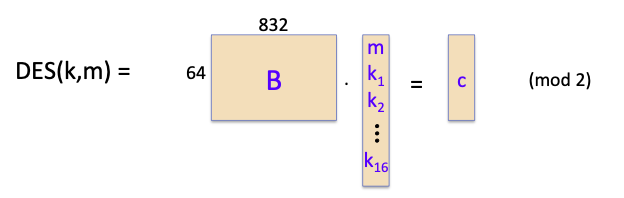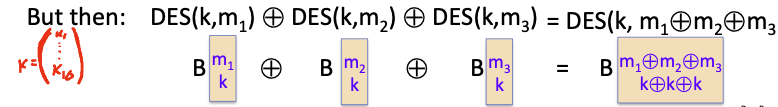## Exhaustive Search Attacks

DES的密钥空间比较小，因此可以使用穷举密钥的方法攻击。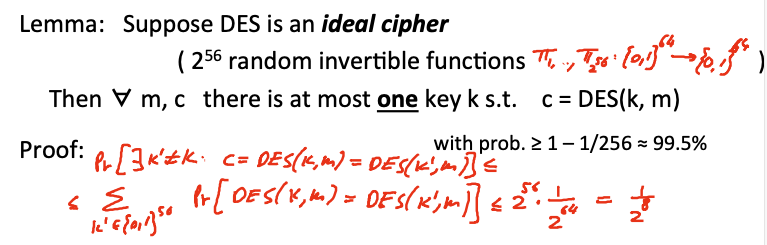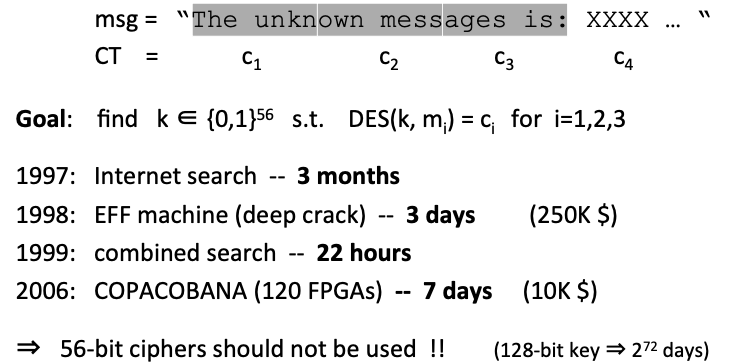### 1: Triple-DES

• Let $\mathrm{E}:\mathrm{K}\times \mathrm{M}\rightarrow \mathrm{M}$ be a block cipher.
• Define $\mathrm{3E}: \mathrm{K}^3\times \mathrm{M}\rightarrow \mathrm{M}$ as $\mathrm{3E}((\mathrm{k_1},\mathrm{k_2},\mathrm{k_3}),\mathrm{m})=\mathrm{E}(\mathrm{k_1},\mathrm{D}(\mathrm{k_2},\mathrm{E}(\mathrm{k_3},\mathrm{m})))$

triple- DES为什么是E、D、E的运算顺序，而不是E、E、E的运算顺序？

Triple- DES的密钥空间大小是 $3\times 56=168$ bits，在运算上会比DES慢三倍，但攻击triple- DES只需要 $O(2^{118})$ 的时间复杂度。（下文介绍的中间相遇攻击）

#### Meet in the middle attack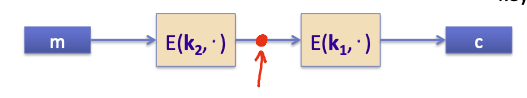double DES的密钥空间为112 bits，但在中间相遇攻击下，穷举范围可以少一半比特。

Attack: M = (m1, …, m10), C = (c1, …, c10)

1. build table and sort on 2nd column.

从double DES 的左边的明文开始，枚举 $k_2$ ，计算 $E(k^i, M)$ 的值，最后按照 $E(k^i, M)$ 的值排序。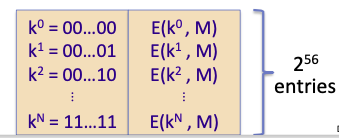2. for all $k\in {0,1}^{56} do:$ test if $D(k,C)$ is in 2nd column.

从double DES的右边的密文开始检测，是否 $D(k,C)$ 存在在上述表中，如果 $E(k^i, M)=D(k,C)$ ，那么就得到了 $(k^i, k)=(k_2, k_1)$

Time = $2^{56} \log \left(2^{56}\right)+2^{56} \log \left(2^{56}\right)<2^{63}<<2^{112}, \quad \text { space } \approx 2^{56}$

### 2: DESX

$\mathrm{E}: \mathrm{K} \times{0,1}^{n} \rightarrow{0,1}^{\mathrm{n}} \text { a block cipher }$

Define EX as $\operatorname{EX}\left(\left(\mathrm{k}{1}, \mathrm{k}{2}, \mathrm{k}{3}\right), \mathrm{m}\right)=\mathrm{k}{1} \oplus \mathrm{E}\left(\mathrm{k}{2}, \mathrm{~m} \oplus \mathrm{k}{3}\right)$

## More attacks on block ciphers

### Attacks on the implementation### Linear and differential attacks

Linear cryptanalysis (overview):

let c = DES(k, m), suppose for random k, m: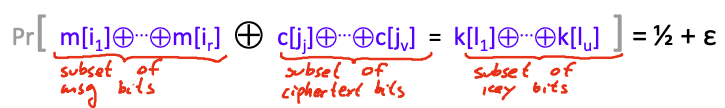Thm: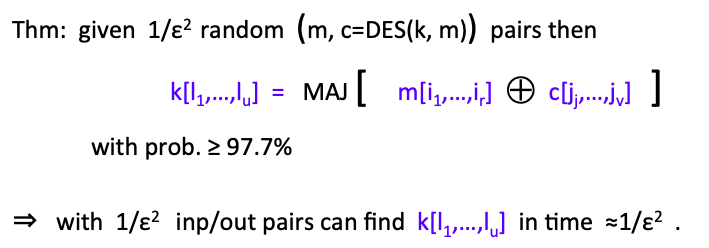Total attack time $\approx 2^{43}<<2^{56}$ with $2^{42}$ random inp/out pairs.

Lesson:

A tiny bit of linearly in $S_5$ lead to a $2^{42}$ time attack.

So don’t design ciphers yourself.

### Quantum attacks# AES

AES的发展过程如图：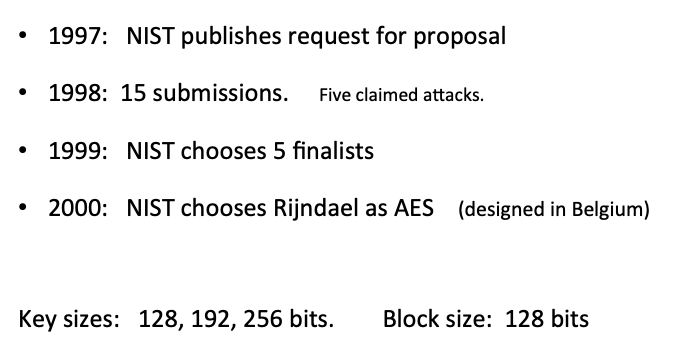AES不是Feistel网络结构的密码，他是一个替换-置换类网络(Subs-Perm network):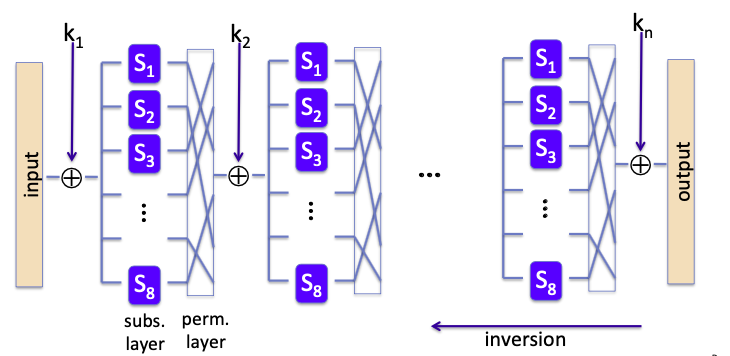## AES-128 schematic

AES-128的结构如下图：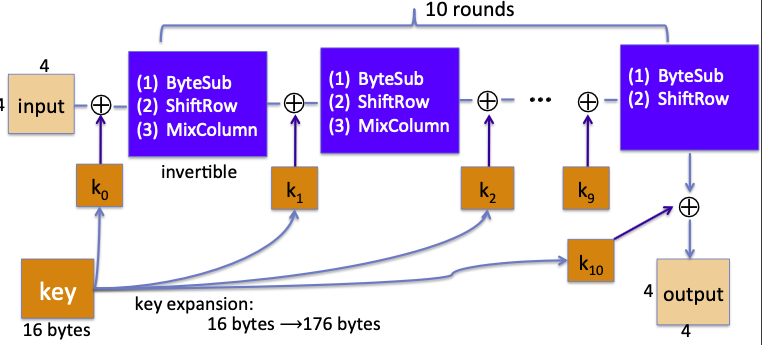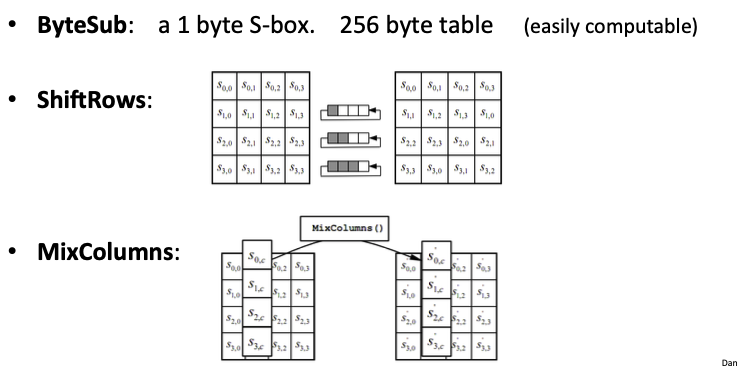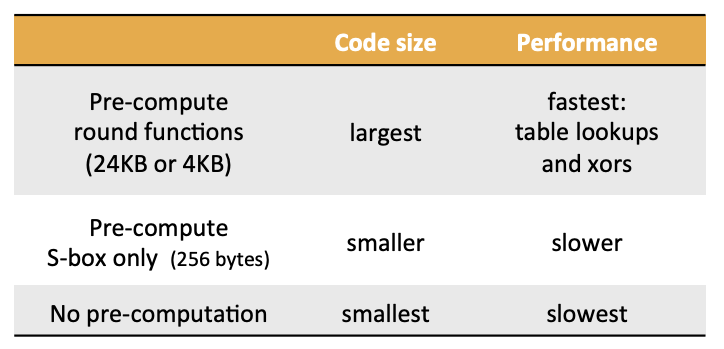## AES in hardware

AES instructions in Intel Westmere:

• aesenc, aesenclast: 执行AES中的一轮运算

• 128-bit registers: xmm1=state, xmm2=round key
• aesenc xmm1, xmm2 ：执行一轮运算，并把计算结果放在xmm1中
• aeskeygenassist: 执行AES的密钥扩展。

## Attacks

AES也存在比暴力搜索密钥空间更好的算法。

• Best key recovery attack: four times better than ex. search [BKR’11]

• Related key apack on AES-256: Given $2^{99}$ inp/out pairs from four related keys in AES-256 can recover keys in time ≈299[BK’09]

# Block ciphers from PRGs

Let G: $K\rightarrow K^2$ be a secure PRG.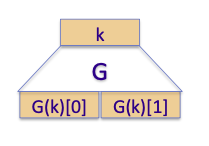Define 1-bit PRF F: $K\times {0,1} \rightarrow K$ as
$$F(k,x\in {0,1})=G(k)[x]$$

Thm: If G is a secure PRG then F is a secure PRF.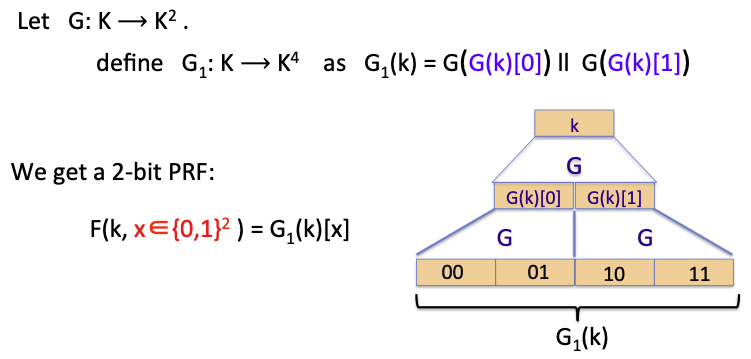Proof: G1 is a secure PRG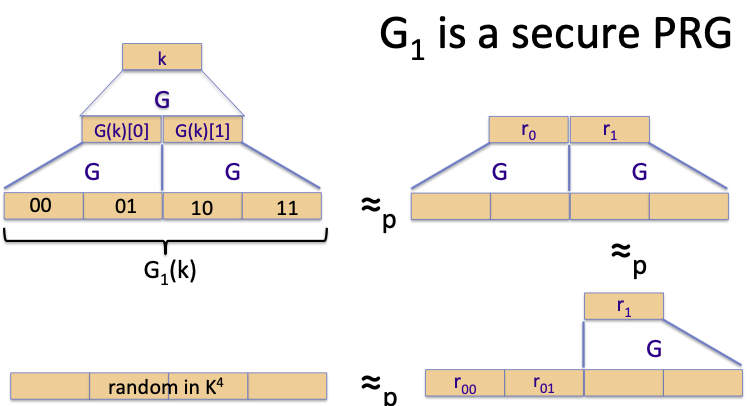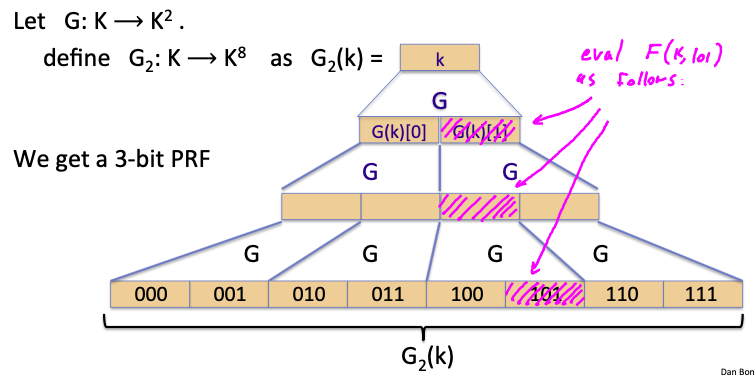GGM PRF就是通过上述的扩展方式得到 ${0,1}^n$ 域上的PRF：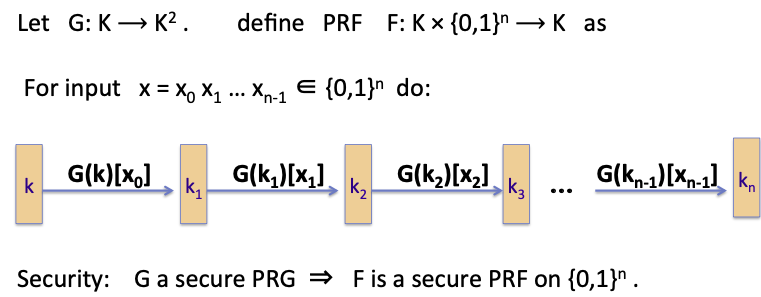Thm:

$f:K\times \{0,1\}^n\rightarrow \{0,1\}^n$ a secure PRF

then, 3-round Feistel $F: K^3\times{0,1}^{2n}\rightarrow {0,1}^{2n}$ a secure PRP.